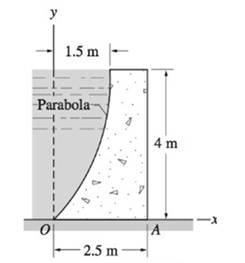# A concrete seawater dam is shown in cross section. Is the dam safe against tipping about edge A ? The mass densities are 24000 kg/m 3 for concrete and 1030 kg/m 3 for seawater.### International Edition---engineerin...

4th Edition
Andrew Pytel And Jaan Kiusalaas
Publisher: CENGAGE L
ISBN: 9781305501607
Chapter 8, Problem 8.118P
Textbook Problem
70 views

## A concrete seawater dam is shown in cross section. Is the dam safe against tipping about edge A? The mass densities are 24000   kg/m 3 for concrete and 1030   kg/m 3 for seawater.To determine

Whether the dam safe against tipping about edge A

### Explanation of Solution

Given information:

Densities of concrete and sea water are 2400kg/m3,1030kg/m3 respectively.

The resultant force P is defined as:

P=pA

In above equation

p - Pressure

A - Area

The weight 'W' of water is defined as:

W=ρg×V

V - Volume

Calculation:

Assume R as the resultant force and N as the normal force.

Assume W1,W2,W3 as the weight of water acting on the dam.

Assume x,y1 as the line of action.

The resultant force R of 1m length of dam

R=12ph=12ρgh2=12(1030kg/m3)(9.81m/s2)(4m)2=80834.4N

The distance y1

y1=43=1.333m

The weight W1 of water

W1=23ρgah=23(1030kg/m3)(9.81m/s2)(1.5m)(4m)=40417.2N

The distance x1

x1=25a=25(1.5)=0.6m

The weight W2 of concrete

W2=gρcbh=(2400kg/m3)(9

### Still sussing out bartleby?

Check out a sample textbook solution.

See a sample solution

#### The Solution to Your Study Problems

Bartleby provides explanations to thousands of textbook problems written by our experts, many with advanced degrees!

Get Started

Find more solutions based on key concepts
What is a relational diagram? Give an example.

Database Systems: Design, Implementation, & Management

What are the units of mass and weight in U.S.?

Engineering Fundamentals: An Introduction to Engineering (MindTap Course List)

Define an object, and provide three examples.

Systems Analysis and Design (Shelly Cashman Series) (MindTap Course List)

Identify reasons to use a virtual machine. List steps for setting up a virtual machine.

Enhanced Discovering Computers 2017 (Shelly Cashman Series) (MindTap Course List)

Which port must be open for RDP traffic to cross a firewall?

Network+ Guide to Networks (MindTap Course List)

If your motherboard supports ECC DDR3 memory, can you substitute non-ECC DDR3 memory?

A+ Guide to Hardware (Standalone Book) (MindTap Course List)